• 1.opengl中缩放使用的函数是glScalef  其原型为void glScalef(GLfloat x,  GLfloat y,  GLfloat z); 该函数表示模型在各轴上进行扩大和缩小。例如：glScalef (1.0, 2.0, 1.0); 表示y坐标值扩大两倍...
1.opengl中缩放使用的函数是glScalef
其原型为void glScalef(GLfloat  x,
GLfloat  y,
GLfloat  z);
该函数表示模型在各轴上进行扩大和缩小。例如：glScalef
(1.0, 2.0, 1.0);
表示y坐标值扩大两倍，这样原本的物体就变长了。
glTranslatef(-50.0f,0.0f,0.0f);glScalef( 1.0f,2.0f,1.0f);//y轴方向放大两倍
glBegin(GL_TRIANGLES);         glVertex3f(0.0f,0.0f, 0.0f);        glVertex3f(50.0f,0.0f, 0.0f);        glVertex3f(0.0f,50.0f, 0.0f);    glEnd();
运行结果图为：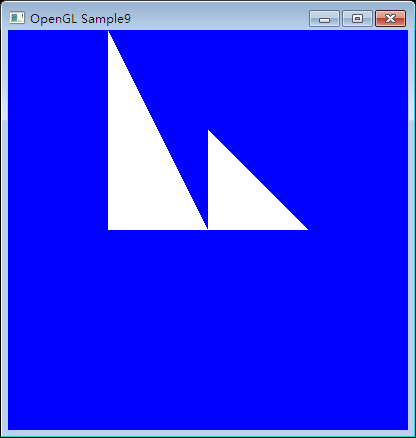2.平移使用glTranslatef函数
其原型为void glTranslatef(GLfloat  x,
GLfloat  y,
GLfloat  z);
例如：glTranslatef(-50.0,0.0,-20.0);表示物体沿x负方向移动50，沿z轴负方向移动20.
需要注意的是，当移动的时候，并不是相对屏幕中心移动，而是相对与当前所在的屏幕移动。
其作用就是将绘点坐标的原点在当前原点的基础上平移一个（x，y，z）向量。

glClear(GL_COLOR_BUFFER_BIT); //清除颜色glBegin(GL_TRIANGLES);     //划线glVertex3f(0.0f,0.0f,0.0f);glVertex3f(50.0f,0.0f,0.0f);glVertex3f(0.0f,50.0f,0.0f);glEnd();

glTranslatef(-50.0f,0.0f,0.0f);//向x的负方向平移50
glBegin(GL_TRIANGLES);         glVertex3f(0.0f,0.0f, 0.0f);        glVertex3f(50.0f,0.0f, 0.0f);        glVertex3f(0.0f,50.0f, 0.0f);   glEnd();
运行结果如下图：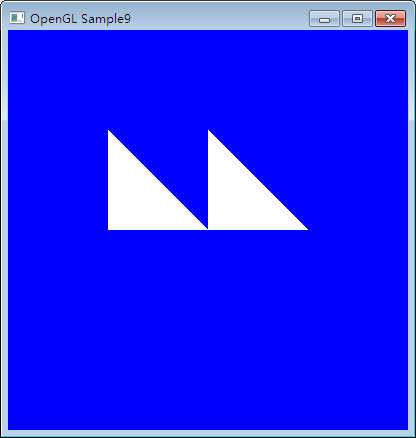3.旋转使用glRotatef函数
其原型为：void glRotatef(GLfloat  angle,
GLfloat  x,
GLfloat  y,
GLfloat  z);
angle表示旋转的角度，(x,y,z)表示转轴。例如：glRotatef(45.0,
0.0, 0.0, 1.0);
表示模型沿着(0,0,1)这个轴旋转45°。
glTranslatef(-50.0f,0.0f,0.0f);
glRotatef(45,0.0f,.0f,1.0f);//按z轴方向旋转45°
glBegin(GL_TRIANGLES);         glVertex3f(0.0f,0.0f, 0.0f);        glVertex3f(50.0f,0.0f, 0.0f);        glVertex3f(0.0f,50.0f, 0.0f);    glEnd();
运行结果为：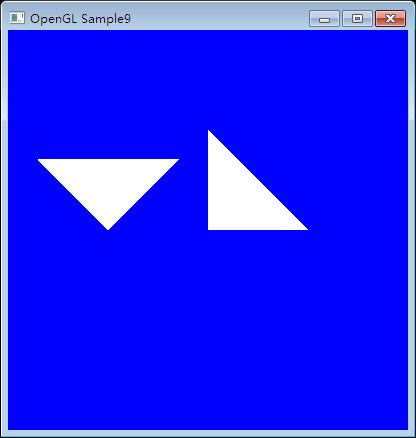展开全文OpenGL
• 在WebGL中，如果我们想实现更高级的动态场景，或者是对物体进行坐标变换，那么旋转平移缩放必不可少。这些变换所涉及的是一些矩阵运算，先从二维平面讲起，再推导至三维空间。下面分别对这几种变换进行一个讲解...
在WebGL中，如果我们想实现更高级的动态场景，或者是对物体进行坐标变换，那么旋转、平移和缩放必不可少。这些变换所涉及的是一些矩阵运算，先从二维平面讲起，再推导至三维空间。下面分别对这几种变换进行一个讲解：

学习交流欢迎加群：789723098，博主会将一些demo整理共享

1 旋转变换

试想一下，如果当一个场景只是静态的，没有动画，那将是多么令人乏味的画面，而旋转变换的加入将会使场景的动画效果更具观赏性。如下图所示，表示点(x, y)绕原点旋转一定角度后到达新的位置，假设原点到想（x, y）半径为R。可参考我另一篇博客的完整示例，绘制一个旋转的彩色立方体：点击打开链接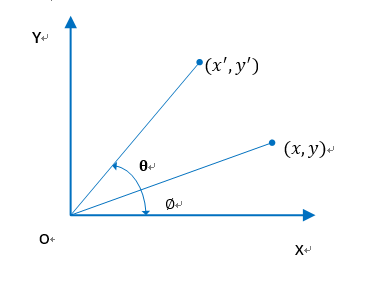图中的关系可由系列式子计算：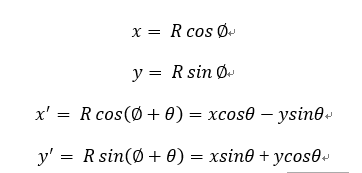由上面的公式，上述坐标的矩阵表达形式为：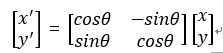将其推广至三维空间，并用其次坐标表示出来，称为旋转矩阵：
绕x轴旋转：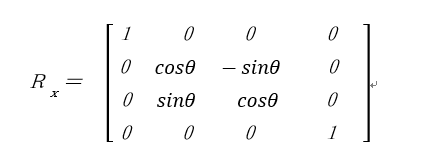绕y轴旋转：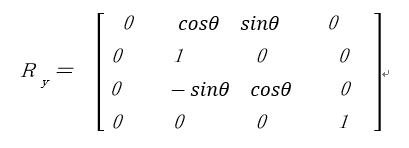绕z轴旋转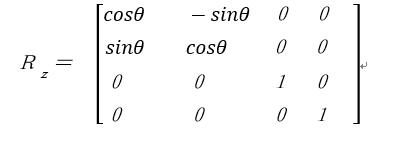2 平移变换

平移是将点沿着指定的方向移动一段距离，即在原有坐标的基础上，再分别给x,y,z加上Tx,Ty,Tz；其示意图如下所示：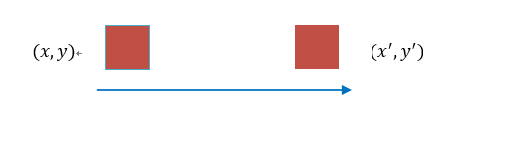平移矩阵如下：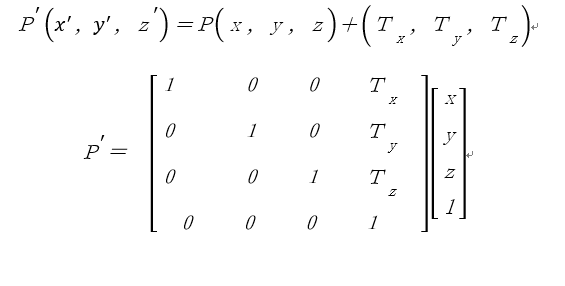3 缩放变换

顾名思义，缩放即对图形的放大缩小，其示意图如下所示：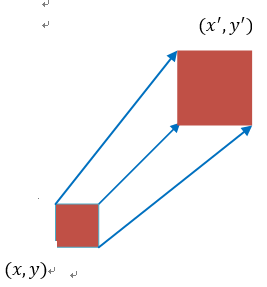缩放的原理是在原有坐标的基础上乘一个缩放因子：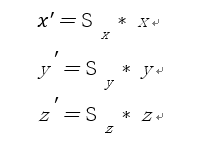其缩放矩阵如下：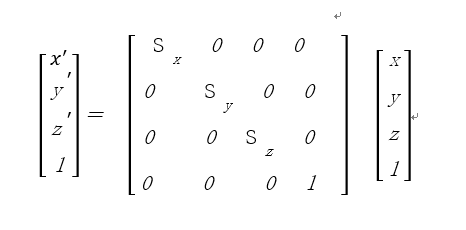以上就是WebGL中常用的三种变换，具体的代码实现在我的另一篇微薄上：点击打开链接。
里面实现了一个可用键盘和鼠标交互的可旋转立方体。

展开全文• 用WebGL绘制的的旋转平移缩放的立方体动画，压缩包内的文件包括HTML、JavaScript可以打开直接运行。
• 笔者在前面的文章主要是针对二维的静态图形进行开发；...本节的内容用到了前面文章提到过的平移缩放旋转变换，如果对这方面还不是很熟悉的同学，可以参考这篇文章：原生webgl学习（三） WebGL中的矩...
笔者在前面的文章主要是针对二维的静态图形进行开发；但有时候我们需要模型动起来，就像真实世界中的一切运动变化一样。场景如果不是动态的，那么可想而知，我们的世界是多么枯燥乏味。为了让我们开发的图形应用看上去更加高大上，这一节笔者将和大家一起做一个动画的例子；本节的内容用到了前面文章提到过的平移、缩放和旋转变换，如果对这方面还不是很熟悉的同学，可以参考这篇文章：原生webgl学习（三） WebGL中的矩阵运算：平移、旋转和缩放。图形动画的根本在于改变图形顶点的位置，打个比方，例如现在的你坐或站在一个位置，咱以这个位置为坐标系原点，下一刻你起身走动，你所处的位置也就发生了变化，跟着组成你身体的顶点位置也相对于坐标系发生了偏移，这种偏移并不是说你身上少了一块肉或者多了一块肉，这只是你在世界坐标系的位置发生了变化而已，如果你持续不断的在走动，就构成了我们所认知的动画，所以动画的本质还是顶点在某个坐标系内位置发生了变化，这种变化是持续不断的。如下图所示：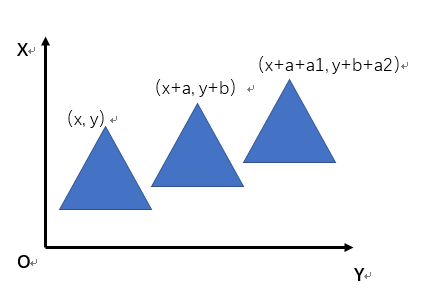如果对笔者的博客上的代码编写方式还不是很熟悉请参考笔者其他的博文：原生 WebGL开发文章目录（持续更新），里面的文章对代码有详细注释和原理解释。说到这里，相信大家已经期待看见动画的效果，笔者只做了一个gif图，呈现了本文demo的动画实现过程，忽略上面的网址（一个录屏软件生成的水印）。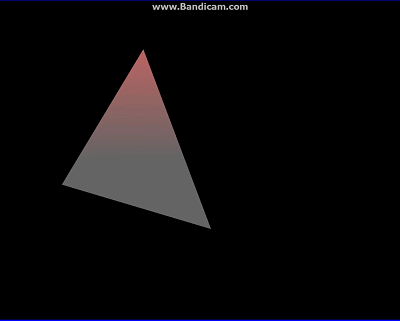接下来我们一起来看一下代码，我们主要看关键功能实现的代码，如果需要完整代码，读者可以自行下载：https://gitee.com/babyogl/learnWebGL，本例代码在文件夹chapter-04中的animate-rotating.html。首先，我们先定义一些与动画相关的变量：

let translate = [100, 400];//初始平移
const TRANSLATE_STEP = 100;//平移幅度
let angle = 0;//旋转角
const ANGLE_STEP = 1.0;//旋转幅度
let scale = [1, 1];//缩放比例

其次，要将定义的平移、旋转和缩放的值传递给着色器：

  let tMatrix = m3.translation(translate, translate);
let rMatrix = m3.rotation(angle);
let sMatrix = m3.scaling(scale,scale);
var matrix = m3.multiply(tMatrix, rMatrix);
matrix = m3.multiply(matrix, sMatrix);

接下来，实现图形旋转、平移和缩放动画的功能：

//实现旋转
let r_last = Date.now();//记录开始时间
function updateAngle(angle) {
let now = Date.now();
let elapsed = now - r_last;
r_last = now;
let newAngle = angle + (ANGLE_STEP * elapsed) / 1000.0;

return newAngle %= 360;
}

//实现平移
let t_last = Date.now();//记录开始时间
function updateTranslate(x, y) {
let now = Date.now();
let elapsed = now - t_last;
t_last = now;

let dx = x + elapsed * TRANSLATE_STEP / 1000;
let tx = dx;

if (tx > 800) {
tx = tx - dx;
scale = 1;
scale = 1;
}
let translate =[tx, y];

return translate;
}

//实现缩放
sx += Math.random() / 200;
sy += Math.random() / 200;
return [sx, sy];

}

最后我们通过resquestAnimationFrame功能实现持续不断的渲染功能，使场景看起来一直在动：

let animate = function() {
angle = updateAngle(angle);
translate = updateTranslate(translate, translate);
drawScene(gl, program);
requestAnimationFrame(animate);
};

整个domo代码码：

<!DOCTYPE html>
<html lang="en">
<meta charset="UTF-8">
<title>旋转动画</title>
<style>
body {
margin: 0px;
overflow: hidden;
}
canvas {
border: 3px solid blue;
display: block;
}
</style>
<body>
<canvas id="rotating" width="1000" height="800"></canvas>
<script type="text/javascript" src="../libs/webgl-utils.js"></script>
attribute vec2 a_position;
uniform vec2 u_resolution;
uniform mat3 u_matrix;
varying vec4 v_color;
void main()
{
vec2 position = (u_matrix * vec3(a_position, 1.0)).xy;
vec2 zeroToone = position / u_resolution;
vec2 clipSpace = zeroToone * 2.0 - 1.0;

gl_Position = vec4(clipSpace * vec2(1, -1), 0, 1);
v_color = vec4(gl_Position.xyz*0.5, 0.6);
}
</script>
#ifdef GL_ES
precision mediump float;
#endif

varying vec4 v_color;
void main()
{
gl_FragColor = v_color.gbra;
}
</script>
<script type="text/javascript">
"use strict";
let translate = [100, 400];//初始平移
const TRANSLATE_STEP = 100;//平移幅度
let angle = 0;//旋转角
const ANGLE_STEP = 1.0;//旋转幅度
let scale = [1, 1];//缩放比例
//3*3矩阵运算
let m3 = {
identity: function() {
return [
1, 0, 0,
0, 1, 0,
0, 0, 1
]
},

translation: function(tx, ty) {
return [
1, 0, 0,
0, 1, 0,
tx, ty, 1
]
},

rotation: function(angle) {
let c = Math.cos(angle);
let s = Math.sin(angle);

return [
c, -s, 0,
s, c, 0,
0, 0, 1
]
},

scaling: function(sx, sy) {
return [
sx, 0, 0,
0, sy, 0,
0, 0, 1
]
},

multiply: function(a, b) {
let a00 = a[0 * 3 + 0];
let a01 = a[0 * 3 + 1];
let a02 = a[0 * 3 + 2];
let a10 = a[1 * 3 + 0];
let a11 = a[1 * 3 + 1];
let a12 = a[1 * 3 + 2];
let a20 = a[2 * 3 + 0];
let a21 = a[2 * 3 + 1];
let a22 = a[2 * 3 + 2];
let b00 = b[0 * 3 + 0];
let b01 = b[0 * 3 + 1];
let b02 = b[0 * 3 + 2];
let b10 = b[1 * 3 + 0];
let b11 = b[1 * 3 + 1];
let b12 = b[1 * 3 + 2];
let b20 = b[2 * 3 + 0];
let b21 = b[2 * 3 + 1];
let b22 = b[2 * 3 + 2];
return [
b00 * a00 + b01 * a10 + b02 * a20,
b00 * a01 + b01 * a11 + b02 * a21,
b00 * a02 + b01 * a12 + b02 * a22,
b10 * a00 + b11 * a10 + b12 * a20,
b10 * a01 + b11 * a11 + b12 * a21,
b10 * a02 + b11 * a12 + b12 * a22,
b20 * a00 + b21 * a10 + b22 * a20,
b20 * a01 + b21 * a11 + b22 * a21,
b20 * a02 + b21 * a12 + b22 * a22,
];
}
};//3*3矩阵
let position = [
0, -100,
150, 125,
-175, 100
];//三角形顶点位置

function main() {
//获取canvas元素，并判断浏览器是否支持webgl
let canvas = document.getElementById('rotating');
let gl = canvas.getContext('webgl', {antialias:true, depth: false});
if(!gl) {
}

//创建、编译并连接着色器

//获取着色器中相关变量的位置
program.pLocation = gl.getAttribLocation(program, 'a_position');
program.reLocation = gl.getUniformLocation(program, 'u_resolution');
program.mLocation = gl.getUniformLocation(program, 'u_matrix');
//创建缓冲区，并绑定
program.pBuffer = gl.createBuffer();
gl.bindBuffer(gl.ARRAY_BUFFER, program.pBuffer);

//动画
let animate = function() {
angle = updateAngle(angle);
translate = updateTranslate(translate, translate);
drawScene(gl, program);
requestAnimationFrame(animate);
};

animate();
}
//画图
function drawScene(gl, program) {
gl.viewport(0, 0, gl.canvas.width, gl.canvas.height);
gl.clearColor(0.0, 0.0, 0.0, 1.0);
gl.clear(gl.COLOR_BUFFER_BIT);
gl.useProgram(program);
gl.enableVertexAttribArray(program.pLocation);
gl.bindBuffer(gl.ARRAY_BUFFER, program.pBuffer);
gl.bufferData(gl.ARRAY_BUFFER, new Float32Array(position), gl.STATIC_DRAW);
gl.vertexAttribPointer(program.pLocation, 2, gl.FLOAT, false, 0, 0);
gl.uniform2f(program.reLocation, gl.canvas.width, gl.canvas.height);
//平移、旋转、缩放运算
let tMatrix = m3.translation(translate, translate);
let rMatrix = m3.rotation(angle);
let sMatrix = m3.scaling(scale,scale);
var matrix = m3.multiply(tMatrix, rMatrix);
matrix = m3.multiply(matrix, sMatrix);

gl.uniformMatrix3fv(program.mLocation, false, matrix);
gl.drawArrays(gl.TRIANGLES, 0, position.length / 2);
}

//实现旋转
let r_last = Date.now();//记录开始时间
function updateAngle(angle) {
let now = Date.now();
let elapsed = now - r_last;
r_last = now;
let newAngle = angle + (ANGLE_STEP * elapsed) / 1000.0;

return newAngle %= 360;
}
//实现平移
let t_last = Date.now();//记录开始时间
function updateTranslate(x, y) {
let now = Date.now();
let elapsed = now - t_last;
t_last = now;

let dx = x + elapsed * TRANSLATE_STEP / 1000;
let tx = dx;

if (tx > 800) {
tx = tx - dx;
scale = 1;
scale = 1;
}

console.log(tx);
// let ty = y + elapsed * TRANSLATE_STEP / 1000;

let translate =[tx, y];

return translate;
}
//实现缩放
sx += Math.random() / 200;
sy += Math.random() / 200;
return [sx, sy];

}
main();
</script>
</body>
</html>

 
展开全文• OpenGL坐标变换　平移缩放旋转 OpenGL有内建的坐标系，事实上OpenGl有两套坐标系，一个坐标系被称为眼睛坐标（eye coordinate system）　简称ECS ,上章讲的就是这个坐标系。 OpenGL还有一套坐标，被称为...
OpenGL坐标变换　平移，缩放与旋转

OpenGL有内建的坐标系，事实上OpenGl有两套坐标系，一个坐标系被称为眼睛坐标（eye coordinate system）　简称ECS 。  OpenGL还有一套坐标，被称为（object
coordinate system）　简称OCS ,而这个才是更为重要的，其实我们用来绘图的正是OCS。
下面是一个平移和缩放例子：

#include<stdio.h>
#include <GL/glut.h>
#include <GL/glext.h>
void makecross(float *color) //在当前OCS的中心画一个十字
{
glBegin(GL_LINES);
glColor3fv(color); //设置当前颜色
glVertex3f(-1,0,0);
glVertex3f(1,0,0);
glVertex3f(0,-1,0);
glVertex3f(0,1,0);
glEnd();
}
void display()
{
float red={1,0,0};
float blue={0,1,0};
float green={0,0,1};
float yellow={1,1,0};

glClearColor(1,1,1,1);
glClear(GL_COLOR_BUFFER_BIT);

makecross(red);
glTranslatef(-0.5,-0.5,0);//坐标平移
makecross(blue);
//glTranslatef(1,0.25,0);
//makecross(green);
//glTranslatef(-0.75,0.75,0);
//glScalef(0.5,0.5,1); //在x,y 上缩小一半
//makecross(yellow);
glFlush();          //更新窗口
}
int main(int argc, char *argv[])
{
glutInit(&argc, argv);
glutInitDisplayMode(GLUT_RGB | GLUT_SINGLE);
glutInitWindowPosition(0, 0);
glutInitWindowSize(600, 600);
glutCreateWindow("OpenGL 3D View");
glutDisplayFunc(display);
glutMainLoop();
return 0;
}
这两个函数中makecross的作用是在坐标中心画一个十字，前面我们知道glVertex使用的是OCS 所以makecross 的作用便是在当前OCS的中心画一个十字，以观察OCS的位置，
glColor3fv(color)的功能于之前我们看到的glColor3f(r,g,b)是一样的，只不过一个是使用一个数组作为参数
2. void glTranslate{fd}(TYPEx, TYPE y, TYPEz);
glTranslatef 是将ＯＣＳ平移至x,y,z 出，也就是在（ｘ，ｙ，ｚ）处建立新的ＯＣＳ，这里要注意这里的参数Ｘ，Ｙ，Ｚ也是OCS坐标
3. void glScale{fd}(TYPEx, TYPE y, TYPEz);
glScalef则是当前OCS的缩放，x,y,z　分别指在三个方向上的放大倍数
说完了缩放和平移，我们来看看旋转，opengl 里的旋转是通过glrotate来实现的，他的本质是将当前矩阵在乘于旋转矩阵，就是将当前的OCS 旋转一个指定的角度
4. void glRotate{fd}(TYPE angle, TYPE x, TYPE y, TYPE z);
glrotate 是将当前OCS绕向量（x,y,z）逆时针旋转angle度　例如我们要讲上例中的图形旋转绕Z轴旋转４５度则可以通过glrotatef(45,0,0,1)来实现。

展开全文opengl
• 有的时候变换可能比较复杂，比如平移之后又旋转旋转之后又平移，又缩放。 直接用公式计算，不但复杂，而且效率低下。这时可以借助变换矩阵和矩阵乘法，将多个变换合成一个。 最后只要用一个矩阵对每个点做一次...矩阵 变换 数学
• 假设绘制顶点的语句为Draw Array，变换的语句（旋转平移缩放）为M，而 M0; M1; M2; Draw Array; 则称对Array先进行M2再进行M1、M0 0x02. 本文基于SharpGL版本的OpenGL(C#版本) l 预备知识： 0x01. gl....
• 仿射变换（Affine Transformation或 Affine Map），又称仿射映射，是指在几何中，一个向量空间进行一次线性变换并接上一个平移，变换为另一个向量空间的过程。它保持了二维图形的“平直性”（即：直线经过变换之后...计算机视觉 opencv
• 自己用了很久对这个几个方法，用了好多次了，但对它们的原理概念都还是没仔细理解清楚，真是太菜了。...然后canvas是可以变换的，我们平移canvas dx，dy，然后draw画图，就相当于把画布拖动一段距离，然后作画，作画android
• imutils是在OPenCV基础上的一个封装，达到更为简结的调用OPenCV接口的目的，它可以轻松的实现图像的平移旋转缩放，骨架化等一系列的操作。 安装： pip install imutils 使用方法： 图像平移 OpenCV中也...opencv 人工智能 图像识别 imutils
• 平移glTranslate&lt;f,d&gt;(tx,ty,tz),对于二维情形，tz=0.0示例如下：glTranslatef(3.0,2.0f,0.0f);旋转 glRotate&lt;f,d&gt;(theta,vx,vy,vz)： theta,vx,vy,vz指定这个旋转物体的矩阵，物体将...
• 平移旋转缩放这个三个是osg矩阵操作中，最常见的操作，下面给出示例以及说明 首先先了解下osg空间方向：  osg方向如左图所示，x轴表示屏幕水平方向，y轴表示和屏幕垂直方向即屏幕里面方向，z轴表示屏幕垂直方向，...
• 因项目需要图片预览，所以做了一个预览的功能，再次备份： xaml代码：  xmlns="http://schemas.microsoft.com/winfx/2006/xaml/presentation"  xmlns:x=... Title="图片预览wpf 图片
• 有的时候变换可能比较复杂，比如平移之后又旋转旋转之后又平移，又缩放。 直接用公式计算，不但复杂，而且效率低下。这时可以借助变换矩阵和矩阵乘法，将多个变换合成一个。 最后只要用一个矩阵对每个点做一次...
• 使用GDI+变换矩阵实现图形的平移缩放旋转 这段时间用GDI+开发一个适量图形绘制软件，类似于CoreDraw，用于教学之用。刚开始用GDI+提供的变换矩阵Matrix实现图形旋转变换，图形的平移缩放则自己通过计算实现。...
• 在unity中，一个平移矩阵是一个单位矩阵与平移参数构成的一个矩阵 把物体的位置转换为矩阵后可得知矩阵中的值与物体位置的关系。 物体设置的位置参数 物体输出的矩阵值 通过图可得知矩阵值的关系和物体位置...
• 有的时候变换可能比较复杂，比如平移之后又旋转旋转之后又平移，又缩放。 直接用公式计算，不但复杂，而且效率低下。这时可以借助变换矩阵和矩阵乘法，将多个变换合成一个。 最后只要用一个矩阵对每个点做一次...a u
• 几何空间变换是图像处理中的最基础的算法，主要包括图像的旋转平移缩放，偏移，组合变换等等，在冈萨雷斯的数字图像处理第三版的第二章就做了相关介绍，最常用的空间坐标变换之一就是仿射变换。虽然仿射变换很...图像处理算法 仿射变换 c++ opencv
• 2.旋转Cow到背面 3.想看清楚Cow面部的细节，改变它大小等等 可能你会说，这还不简单，通过操作相机就好了。操作相机，使得相机的空间位置发生了变化，但对三维物体的空间位置并没有改变，要想改变模型的空间位置，...VTK Transform 空间变换
• 1.translate(x,y):平移，将画布的坐标原点向左右方向移动x，向上下方向移动y.canvas的默认位置是在（0,0）.  例子：画布原点假如落在（1，1），那么translate（10，10）就是在原点（1，1）基础上分别在x轴、y轴移动...
• 在图形显示中，图形变换只有平移旋转缩放三种情况。他们都是通过矩阵运算获得的。webgl
• 旋转平移、伸缩与剪切 绕任意轴旋转 注：Coordinate systems and frames，本文译为坐标系与广义坐标系。 一、坐标系与广义坐标系 1.1 坐标系旋转变换 向量 v∈R3v \in R^3v∈R3 可以用一组基向量v1,v2,v3v_1, v_...
• 这里实现的是多点画多边形，然后把这个多边形进行二维的变换。 首先，多点画多边形，为了方便起见，我直接调用...假如我要平移a到b的位置： 那么操作就是：把这个多边形的n个顶点从一个位置移动到另外一个位置，然后变换 多边形
• 若该文为原创文章，未经允许不得转载 原博主博客地址：https://blog.csdn.net/qq21497936 ... 目录 前言 变换模型的添加与删除 添加变换模型 ...平移 缩放 旋转 扩展：OSG中正确的矩阵变换级联顺序 操作过程 ...变换
• （1）平移 我们能通过与下面的矩阵相乘把向量(x, y, z, 1)沿x轴移动px个单位，沿y轴移动py 个单位， 沿z 轴移动pz个单位。 只需要用位置向量(x, y, z, 1)和矩阵做乘法运算即可，对应的矩阵是： DX中对应的平移函数...
• 平移translate 翻转flip 图像的仿射变换Affine transformation基本图像处理1. 缩放scale缩放通过cv2.resize()实现函数说明：cv2.resize(src, dsize[, dst[, fx[, fy[, interpolation]]]]) -> dst 参数说明： src -图像处理 opencv python
• 在上一篇文章中我们介绍了如何使用OpenGL ES预览视频，在文章的末尾提到如果渲染视频的窗口宽高比和视频宽高比不一致会导致视频拉伸，这篇文章将会介绍如何通过视频的缩放来解决这个问题。 我们希望当视频的比例和...openglmatlab 图像处理...